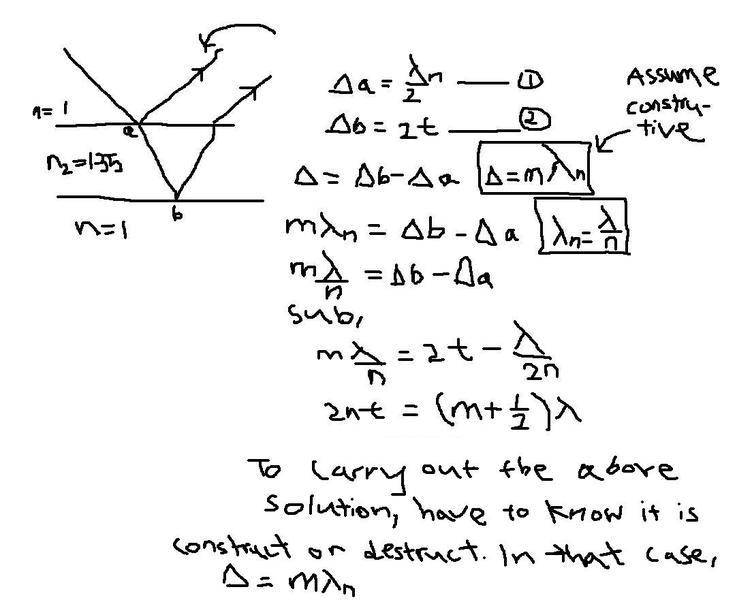# Interference in Thin Films

killerfish
Hi guys,

i have problem with Interference in Thin Films, i tried worked the solution not sure it is correct. Hope someone can help me check? Also there is some part on the topic i dont quite follow, 2t is the extra distance travelled inside the film, then is 2t is also rough estimate of wavelenth?

## Homework Statement

A soap bubble (n = 1.33) is floating in air. If the thickness of the bubble wall is 115 nm,
what is the wavelength of the light that is most strongly reflected?

## The Attempt at a Solution

the worked solution by me is in attachment.Thank you.

## Answers and Replies

nickjer
After a quick look through, it looks fine to me.

Also, 2t is related to the wavelength from the equation you just worked out. It is more directly related to the wavelength of the light inside of medium, n=1.33. But not exactly sure what you are asking.

killerfish
sry for the late reply...

1. for example, if the system give destructive result, i should sub in (m + 1/2)$$\lambda$$n into $$\Delta$$ at the third line of equation ?

2. and you mentioned the 2t is relate to the wavelength, then we actually can use that to find the wavelength entered into the thin film using simple trigo rule?

Last edited:
nickjer
1. Yes, for destructive interference the path length difference needs to be that.

2. You already solved it, so not sure what else you are trying to do here.